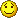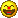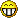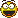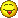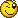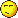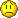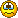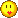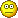... Mek's creations and more

## Home magnetotherapy device

In Czech magazine Amatérske rádio (issue number PE05/2012), there was a simple construction of DIY magnetic therapy device. The author stated that it cured his tennis elbow, and made more pieces of this device for his friends (also doctors) and got only positive feedback. I liked that frequency can be regulated and that all the components can be mined from old TV, or amateurs probably have these in their stock.

I took the schematic and tried it out on a breadboard. It worked on first try. I used a decoupling coil from old TV as application coil. It has ferrite core, inductance 15 mH and resistance 5 ohms. The impulses can be felt after moving a permanent magnet close to the coil, as can be seen in the video below. This is also a simple way to verify the device is actually working.

I enhanced the device with a frequency meter and shutdown timer. Of course, AVR ATmega8 was the right one to do the job. In the video and pictures below, frequency is shown on the red display and shutdown timer is shown on the green display. There is also a red LED which blinks in the rhythm of impulses, and a potentiometer to regulate frequency. Shutdown timer can be set up by four buttons:  +1min, -1min, +5min, -5min. Frequency range is 1-80 Hz. According to this page, that frequency range should suffice.

Following is the schematic. If you don't require frequency meter and shutdown timer, you can omit the bottom part with AVR, MOSFET and relay. The MOSFET can be probably any N enhancement-mode transistor. I had a 2SK2962 at hand but I believe 2N7000 would also work. It should work also with a BJT NPN transistor, but then you must add a base resistor (not tried this option).

Display should be a common anode one.

Beware - transistor TIP122 is heating up with higher frequencies, I recommend to put it on a small cooler.

Power supply is 5V DC from a wall adapter. Current consumption in peaks (when current flows into the coil) can be as high as 1 A.As I already wrote, frequency measurement and shutdown timer is done by MCU Atmel ATmega8. Instead of a crystal, internal RC oscillator is used and it is running at 8 MHz. This spared two pins, because no high precision of CPU clock is required. When programming the MCU, fuse bits must be set like this:

• HIGH: 0xD9
• LOW: 0xE4

And this is the complete program.

``#include "hw.h"#include <avr/io.h>#include <avr/interrupt.h>#include <util/delay.h>#include <stdio.h>#define _BV(bit) (1 << (bit)) // _BV macro is not defined for atmega8 so define it here// BTNx is 1 if respective button is pressed#define BTN0() (bit_is_clear(PIND, 0))#define BTN1() (bit_is_clear(PIND, 1))#define BTN2() (bit_is_clear(PIND, 2))#define BTN3() (bit_is_clear(PIND, 3))#define SEC_MIN 0#define SEC_MAX 99 * 60 + 59volatile unsigned char freq1 = 0; // second digit of frequency displayvolatile unsigned char freq10 = 0; // first digit of frequency displayvolatile unsigned char activeseg = 0; // indicates active display segment (used for multiplexing)volatile unsigned int sec = 20 * 60; // default timer countdown: 20min and counting downvolatile unsigned int btn0cycles = 0; // these 4 are here to support one-time push and push-and-hold on the buttonsvolatile unsigned int btn1cycles = 0;volatile unsigned int btn2cycles = 0;volatile unsigned int btn3cycles = 0;#define _s_A 0#define _s_B 1#define _s_C 2#define _s_D 3#define _s_E 4#define _s_F 5#define _s_G 6#define _s_dot 7const unsigned char segs[] ={	_BV(_s_A) | _BV(_s_B) | _BV(_s_C) | _BV(_s_D) | _BV(_s_E) | _BV(_s_F), // 0	_BV(_s_B) | _BV(_s_C), // 1	_BV(_s_A) | _BV(_s_B) | _BV(_s_D) | _BV(_s_E) | _BV(_s_G), // 2	_BV(_s_A) | _BV(_s_B) | _BV(_s_C) | _BV(_s_D) | _BV(_s_G), // 3	_BV(_s_B) | _BV(_s_C) | _BV(_s_F) | _BV(_s_G), // 4	_BV(_s_A) | _BV(_s_C) | _BV(_s_D) | _BV(_s_F) | _BV(_s_G), // 5	_BV(_s_A) | _BV(_s_C) | _BV(_s_D) | _BV(_s_E) | _BV(_s_F) | _BV(_s_G), // 6	_BV(_s_A) | _BV(_s_B) | _BV(_s_C), // 7	_BV(_s_A) | _BV(_s_B) | _BV(_s_C) | _BV(_s_D) | _BV(_s_E) | _BV(_s_F) | _BV(_s_G), // 8	_BV(_s_A) | _BV(_s_B) | _BV(_s_C) | _BV(_s_D) | _BV(_s_F) | _BV(_s_G), // 9};void addOrSubtractSeconds(int num){	int result = sec + num;	if (result >= SEC_MAX)	{		result = SEC_MAX;	}	else if (result <= SEC_MIN)	{		result = SEC_MIN;	}		sec = result;}int main(void){	unsigned int i = 0;	DDRB = 0xFF; // PD0-7 are outputs	DDRC = 0b00111111; // PC0-PC5 are outputs, PC6-PC7 are inputs (unused)	DDRD = 0b10000000; // PD7: output to drive relay, PD0-PD3: buttons input		// show empty display at the beginning	PORTB = 0xFF;	PORTC = 0xFF;		// PORTB: segments	// PORTC: common anodes		// TIMER0 configuration	TCCR0 |= _BV(CS01) | _BV(CS02); // configure clock source for TIMER0 on pin T0		// TIMER1 configuration	TIMSK |= _BV(TOIE1); // enable overflow interrupt	TCCR1B |= _BV(CS11); // configure prescaler to 8 and start timer	// TIMER2 (display multiplexing) configuration	TIMSK |= _BV(TOIE2); // enable overflow interrupt	TCCR2 |= _BV(CS21); // configure prescaler to 8 and start timer	sei(); // enable global interrupts		// turn on relay initially	PORTD |= _BV(7);			while (1)	{		// maximum that this code detects, is 255 Hz, then timer overflows - this is not handled here as I won't need it (maximum real world frequency is 99 Hz)		TCNT0 = 0;		_delay_ms(1000);		i = TCNT0;		freq1 = i % 10;		freq10 = i / 10;				// timer handling		if (sec != 0)		{			addOrSubtractSeconds(-1);			// turn on relay (useful if time was raised by buttons)			PORTD |= _BV(7);		}		else		{			// turn off relay			PORTD &= ~_BV(7);		}	}}// button handlingISR(TIMER1_OVF_vect){	if (BTN0())	{		if (btn0cycles == 0 || btn0cycles > 10)		{			addOrSubtractSeconds(60);		}				btn0cycles++;	}	else if (BTN1())	{		if (btn1cycles == 0 || btn1cycles > 10)		{			addOrSubtractSeconds(-60);		}				btn1cycles++;	}	else if (BTN2())	{		if (btn2cycles == 0 || btn2cycles > 10)		{			addOrSubtractSeconds(60 * 5);		}			btn2cycles++;	}	else if (BTN3())	{		if (btn3cycles == 0 || btn3cycles > 10)		{			addOrSubtractSeconds(-60 * 5);		}			btn3cycles++;	}		if (!BTN0())	{		btn0cycles = 0;	}		if (!BTN1())	{		btn1cycles = 0;	}		if (!BTN2())	{		btn2cycles = 0;	}		if (!BTN3())	{		btn3cycles = 0;	}}// display multiplexingISR(TIMER2_OVF_vect){	if (activeseg == 5)	{		activeseg = 0;	} else {		activeseg++;	}	switch (activeseg)	{		case 0:			PORTB = ~segs[freq10];			PORTC = 1;		break;		case 1:			PORTB = ~segs[freq1];			PORTC = 2;		break;		case 2:			// sec1			PORTB = ~segs[sec % 10];			PORTC = 4;		break;		case 3:			// sec10			PORTB = ~segs[(sec % 60) / 10];			PORTC = 8;			// blinking double dot			if ((sec & 1) == 0 || sec == 0)			{				PORTB &= ~_BV(_s_dot);		    } else {				PORTB |= _BV(_s_dot);			}		break;		case 4:			// min1			PORTB = ~segs[(sec / 60) % 10];			PORTC = 16;			// blinking double dot			if ((sec & 1) == 0 || sec == 0)			{				PORTB &= ~_BV(_s_dot);		    } else {				PORTB |= _BV(_s_dot);			}		break;		case 5:			// min10			PORTB = ~segs[sec / 600];			PORTC = 32;		break;	}}``

I built the device into a plastic casing. It is pretty tight inside and I had to make a few compromises when designing the PCB. I believe the PCB will be easily designed by everyone according to plastic casing, so I am not publishing it here.

I don't have any personal experience with this device yet, but I like to have it handy. I don't get spine or joint pain very often, but will try this device when I do.

Unfortunately, I cannot find out any technical information about magnetic therapy on the internet. It's full of that marketing and sales crap. Especially answers to questions like these:

• what should an optimal application coil look like (core, turn number, inductance, resistance)
• which frequencies work best for which diseases
• how long and how often to apply magnetic therapy

It seems that the companies producing these devices for hundreds to thousands of dollars, protect these information as their know-how.

If you build this device, I will be glad to receive your opinion which I can also publish here. In case of unclarity or problems while building it I will be glad to help, just use the comment form below.